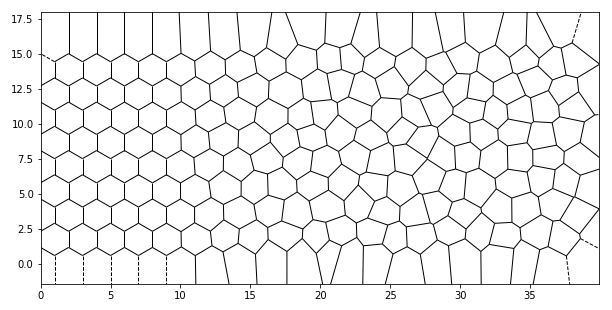Somewhere I saw a picture of a high-performance computer, where the doors would have a hexagonal pattern that would "degrade" into a irregular Voronoi pattern as ventilation holes.

That can be replicated quite easily with a short python script! If you like, you can then use my Voronoi pattern generator for FreeCAD to build such ventilation holes yourself.

The python code is pretty straight forward. First, you need a couple of libraries:

```import numpy as np
from scipy.spatial import Voronoi, voronoi_plot_2d
import matplotlib.pyplot as plt
from itertools import count
```

Next up, we generate a couple of points that are the centers of tiled hexagons:

```def hex_pattern(a: float = 1, nx: int = 20, ny: int = 10, start: int = 1) -> np.array:
"""
Create a pattern with nx times ny cells
:param float d: edge length of the hexagon
:param int nx: number of cells in x-direction
:param int ny: number of cells in y-direction
:param int start: if the offset is on even (0) or odd (1) rows
"""
points = np.empty((nx * ny, 2), dtype=float)
if start not in (0, 1):
raise ValueError('start must be either 0 or 1')
if nx < 1:
raise ValueError("there must be a minimum of 1 cells in x")
if ny < 1:
raise ValueError("there must be a minimum of 1 cells in y")
if a <= 0:
raise ValueError("Edge length of hexagon must be positive and non-zero")
dx = 2 * a
dy = np.sqrt(3) * a
i = count()
for y in range(ny):
offset = a if y % 2 == start else 0
for x in range(nx):
points[next(i)] = dx * x + offset, dy * y
return points

points = hex_pattern()
```

Now, we want some randomness in there. We set up several functions to add the randomness:

```def linear(x: np.array, x0: float = -0.5, x1: float = 0) -> np.array:
"""
Linear gradient between x0 and x1, values lower x0 are set to 0,
higher than x1 to 1
"""
x = x.copy()
zeros = x < x0
ones = x > x1
z = x[(x >= x0) & (x <= x1)]
r = z.max() - z.min()
z = (z - z.min()) / r
x[(x >= x0) & (x <= x1)] = z
x[zeros] = 0
x[ones] = 1
return x

def norm_interval(x: np.array, a: float = -1, b: float = 1) -> np.array:
"""Normalize values to the new interval [a, b]"""
r = x.max() - x.min()
return (((x - x.min()) / r) * (b - a)) + a

def add_random(p: np.array, fun, k: float = 1, coord: int = 0) -> np.array:
"""Add a random displacement to a point with a threshold function"""
r = k * np.random.random(p.shape)
# The idea here is to scale the x (or y) values of the points to [-1, 1],
# then give it to the threshold function, which returns scaling factors [0, 1]
c = fun(norm_interval(p[:, coord])).reshape(-1, 1)
return p + r * c

```

Now, we can use the Voronoi function to generate the cells (or you use the points directly in the FreeCAD script):

```vor = Voronoi(points)

plt.figure(figsize=(10,5))
voronoi_plot_2d(vor, plt.gca(), show_points=False, show_vertices=False)
plt.axis('equal')
plt.xlim(np.min(points[:, 0]), np.max(points[:, 0]))
plt.ylim(np.min(points[:, 1]), np.max(points[:, 1]))
plt.show()
```

And here we are: